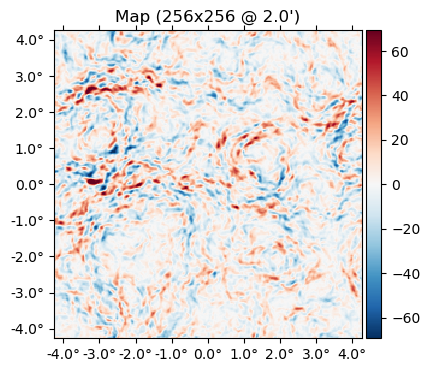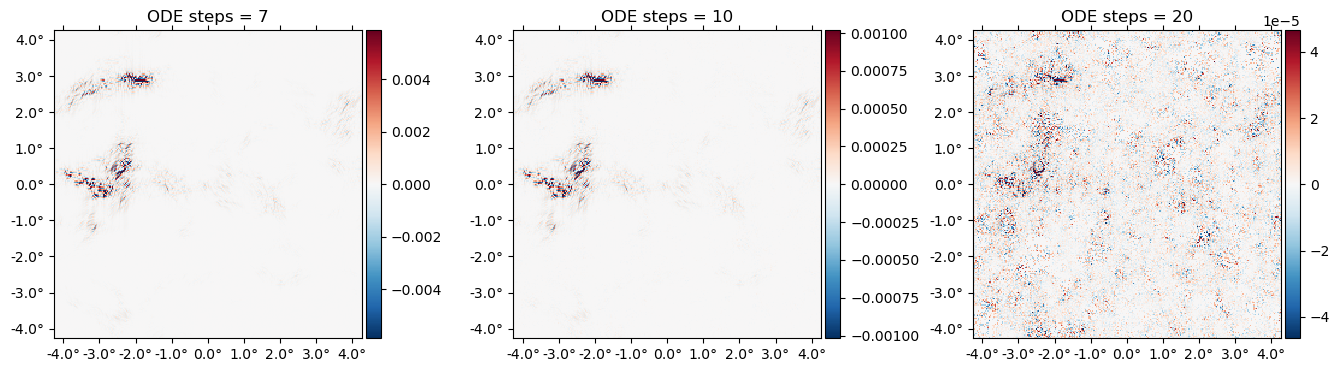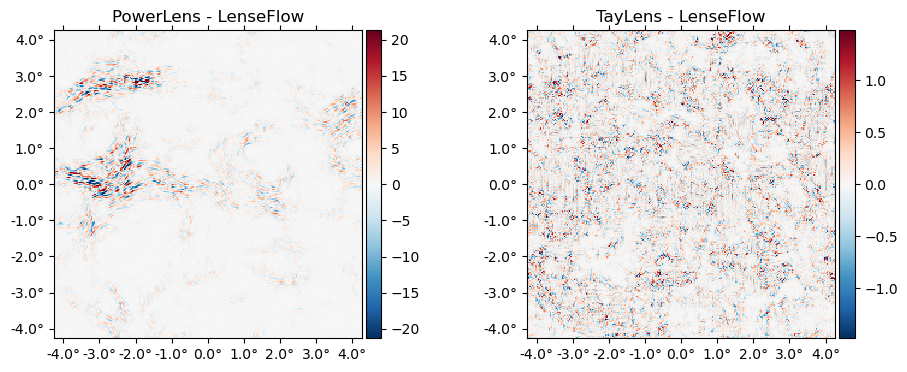# Lensing a flat-sky map

using CMBLensing, PythonPlot
    CondaPkg Found dependencies: /home/cosmo/.julia/packages/PythonCall/qTEA1/CondaPkg.toml
CondaPkg Found dependencies: /home/cosmo/.julia/packages/PythonPlot/KcWMF/CondaPkg.toml
CondaPkg Found dependencies: /home/cosmo/CMBLensing/CondaPkg.toml
CondaPkg Dependencies already up to date

First we load a simulated unlensed field, $f$, and lensing potential, $\phi$,

(;ds, f, ϕ) = load_sim(
θpix  = 2,       # size of the pixels in arcmin
Nside = 256,     # number of pixels per side in the map
T     = Float32, # Float32 or Float64 (former is ~twice as fast)
pol   = :I,      # :I for Intensity, :P for polarization, or :IP for both
);

We can lense the map with LenseFlow,

f̃ = LenseFlow(ϕ) * f;

And flip between lensed and unlensed maps,

animate([f,f̃], fps=1)

The difference between lensed and unlensed,

plot(f-f̃);CMBLensing flat-sky Field objects like f or ϕ are just thin wrappers around arrays. You can get the underlying data arrays for $I(\mathbf{x})$, $Q(\mathbf{x})$, and $U(\mathbf{x})$ with f[:Ix], f[:Qx], and f[:Ux] respectively, or the Fourier coefficients, $I(\mathbf{l})$, $Q(\mathbf{l})$, and $U(\mathbf{l})$ with f[:Il], f[:Ql], and f[:Ul],

mapdata = f[:Ix]
256×256 view(::Matrix{Float32}, :, :) with eltype Float32:
-7.90697  -23.8604    -29.1573    …   70.5152    49.912      17.6294
2.50834   -9.63395   -11.5977        68.4896    49.1049     22.7042
22.8893     8.41276     1.72679       70.4105    58.9074     41.3435
47.4525    31.7446     17.571         74.4612    73.2948     64.1024
71.7069    60.7114     41.0848        73.8759    80.5367     79.0025
87.1937    85.9815     66.443     …   66.3927    76.5174     81.9043
86.6302    96.0922     85.5745        49.9633    62.753      72.8137
82.0516    99.1671     97.7158        26.8173    40.9707     60.1828
75.6491    93.7056     98.1103         2.02564   15.9333     44.6318
61.7563    75.1204     82.4124       -13.8801    -0.509251   32.3792
43.6967    53.2207     63.0691    …  -18.3006    -6.49731    21.4504
16.8759    28.862      41.7566       -20.8806   -13.8449      1.62936
-15.8104     1.26158    19.1784       -26.234    -29.2596    -26.4819
⋮                                ⋱                           ⋮
66.587     45.9522     35.7121        34.6397    51.9126     71.3863
70.8248    54.5411     45.0611    …   13.7916    41.2712     68.8845
61.7769    49.4201     41.3269        12.2368    36.6275     58.6489
47.8602    33.9526     24.7586        26.0094    42.4797     52.4413
39.4095    13.9488     -0.533657      44.745     58.2708     57.4963
33.1774    -0.874786  -15.5843        52.4656    68.094      61.8748
23.0361    -8.15075   -17.4119    …   42.8998    58.0253     51.8293
6.22507  -11.3786    -10.0727        23.0653    33.0222     26.9182
-9.62298  -17.1808     -7.76838        8.90132   14.0551      5.83744
-22.4585   -33.382     -25.1453        17.5381    14.6349     -2.58727
-25.6234   -45.127     -46.49          43.547     31.326       2.40708
-17.3364   -38.313     -46.5364    …   64.2141    47.1561     12.6503

If you have your own map data in an array you'd like to load into a CMBLensing Field object, you can construct it as follows:

FlatMap(mapdata, θpix=3)
65536-element 256×256-pixel 3.0′-resolution LambertMap{SubArray{Float32, 2, Matrix{Float32}, Tuple{Base.Slice{Base.OneTo{Int64}}, Base.Slice{Base.OneTo{Int64}}}, true}}:
-7.906971
2.508335
22.88932
47.45249
71.70686
87.19371
86.63016
82.0516
75.649055
61.75634
43.696697
16.875917
-15.810358
⋮
71.38629
68.88454
58.648865
52.44133
57.496338
61.874783
51.82929
26.918247
5.8374405
-2.5872736
2.407084
12.650339

For more info on Field objects, see Field Basics.

## Inverse lensing

You can inverse lense a map with the \ operator (which does A \ b ≡ inv(A) * b):

LenseFlow(ϕ) \ f;

Note that this is true inverse lensing, rather than lensing by the negative deflection (which is often called "anti-lensing"). This means that lensing then inverse lensing a map should get us back the original map. Lets check that this is the case:

Ns = [7 10 20]
plot([f - (LenseFlow(ϕ,N) \ (LenseFlow(ϕ,N) * f)) for N in Ns],
title=["ODE steps = $N" for N in Ns]);A cool feature of LenseFlow is that inverse lensing is trivially done by running the LenseFlow ODE in reverse. Note that as we crank up the number of ODE steps above, we recover the original map to higher and higher precision. ## Other lensing algorithms We can also lense via: • PowerLens: the standard Taylor series expansion to any order: $$$f(x+\nabla x) \approx f(x) + (\nabla f)(\nabla \phi) + \frac{1}{2} (\nabla \nabla f) (\nabla \phi)^2 + ...$$$ • TayLens (Næss&Louis 2013): like PowerLens, but first a nearest-pixel permute step, then a Taylor expansion around the now-smaller residual displacement plot([(PowerLens(ϕ,2)*f - f̃) (Taylens(ϕ,2)*f - f̃)], title=["PowerLens - LenseFlow" "TayLens - LenseFlow"]);## Benchmarking LenseFlow is highly optimized code since it appears on the inner-most loop of our analysis algorithms. To benchmark LenseFlow, note that there is first a precomputation step, which caches some data in preparation for applying it to a field of a given type. This was done automatically when evaluating LenseFlow(ϕ) * f but we can benchmark it separately since in many cases this only needs to be done once for a given$\phi$, e.g. when Wiener filtering at fixed$\phi\$,

using BenchmarkTools
@benchmark precompute!!(LenseFlow(ϕ),f)
BenchmarkTools.Trial: 236 samples with 1 evaluation.
Range (min … max):  17.417 ms … 30.055 ms  ┊ GC (min … max):  0.00% … 14.41%
Time  (median):     22.042 ms              ┊ GC (median):    18.61%
Time  (mean ± σ):   21.230 ms ±  2.107 ms  ┊ GC (mean ± σ):  14.70% ±  8.03%

▅█▂
▃▇█▄▃▂▁▁▁▁▁▁▁▁▁▁▁▁▁▁▁▁▁▂▃▇███▆▅▃▁▁▁▁▁▃▁▁▁▁▁▁▁▁▁▂▂▁▁▁▁▁▁▁▁▁▂ ▃
17.4 ms         Histogram: frequency by time        27.5 ms <

Memory estimate: 62.99 MiB, allocs estimate: 804.

Once cached, it's faster and less memory intensive to repeatedly apply the operator:

@benchmark Lϕ * f setup=(Lϕ=precompute!!(LenseFlow(ϕ),f))
BenchmarkTools.Trial: 91 samples with 1 evaluation.
Range (min … max):  32.274 ms … 39.005 ms  ┊ GC (min … max): 0.00% … 10.87%
Time  (median):     33.371 ms              ┊ GC (median):    0.00%
Time  (mean ± σ):   34.092 ms ±  1.836 ms  ┊ GC (mean ± σ):  2.34% ±  4.44%

▁   █   ▁
▃▃▇██▆▆██▇▆██▃▇▆▃▆▃▄▁▁▁▁▃▁▁▁▁▁▁▁▁▁▁▁▁▁▁▁▁▁▁▁▃▃▁▃▄▁▁▄▃▄▄▁▃▃▄ ▁
32.3 ms         Histogram: frequency by time        38.4 ms <

Memory estimate: 16.13 MiB, allocs estimate: 441.

Note that this documentation is generated on limited-performance cloud servers. Actual benchmarks are likely much faster locally or on a cluster, and yet (much) faster on GPU.